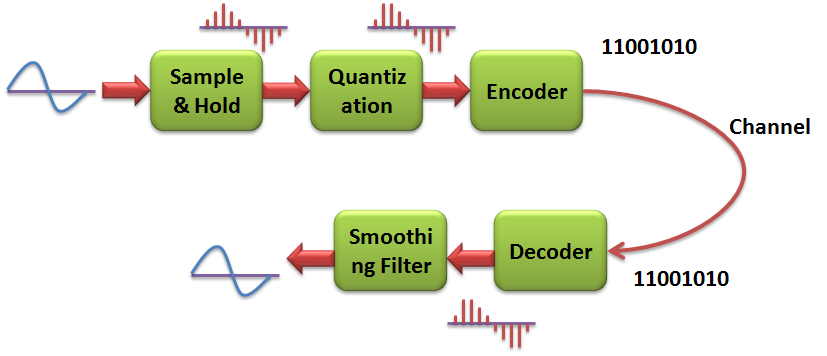## Wednesday, April 3, 2013

### Automatic Room light Controller using OpAmp:

It is more important to save power. here is the one way to save the power by automatic controlling of room lights. This is also an application of OpAmp based comparator. This is based on the light intensity in the room, here we use LDR(light dependent resistor) who's resistance decreases with increase in room light intensity. There is a choice that you can change the threshold level to your desired level so that it can operate at that intensity level. It does not require any microcontroller or any other programmable devices.

components procured:
>LDR
>OpAmp 741
>resistor 10k
>BC547 transistor
>SPDT relay 12V
>1N4001 diode
>battery and a bulb

here i connected the LDR output to the inverting terminal and a threshold voltage of 1.5v is connected to the non-inverting terminal. so that whenever the light intensity increases the LDR output will decrease and if it is less than the threshold the output of the opamp will be +Vsat=+5v.This will trun on the BC547 so that relay will be connected as shown here, bulb will not glow
when the room light intensity decreases LDR resistance will decrease and voltage across the resistor goes high. when it is greater than the threshold opamp output goes to -Vsat=-5v. then the BC547 will goes to the cutoff region (OFF state). so the light will glow.
VLDR < 1.5v ; transistor OFF; light ON;               SPDT Relay

### ADC and DAC using MATLAB

Here we add some Gaussian noise to the input sine wave and then will will convert that to digital signal. This digital signal sampled data will be used in Modelsim. In the modelsim we develop moving average filter using VHDL, this will filters the sampled data and writes into another file. Using the new updated sampled data we will regenerate the Analog signal.clear all;
close all;
clc;
fs=500000;
% taking sampling frequency as 500kHz
fm=10000;
% input signal frequency 10kHz
t=1:200;     % displaying 200 samples
x=5*cos(2*pi*(fm/fs)*t);
%input sinusoidal signal
z=awgn(x,1);
% adding white Gaussian noise to the input signal with S/N=1
h=1:1000;
plot(t,x,'g','LineWidth',2);
% plotting input signal
hold on;
plot(t,z,'r','linewidth',1.5);
% plotting noisy signal
hold on;
stem(t,z);
hold on;
Vd=-5:0.0390625:5; % step size =0.0390625, when n=8 bits
for i=1:256
Vdelta(i)=(Vd(i)+Vd(i+1))/2;
% Quantization levels
end

i=0:255;
binary= dec2bin(i);
% decimal to binary conversion
% Quantization of input signal

for i=1:200
for j=1:256
if(z(i)< Vd(1))
z(i) = Vdelta(1);
end
if (z(i) > Vd(257))
z(i) = Vdelta(256);
end
if(z(i) <= Vd(j+1) && z(i) >= Vd(j))
z(i) = Vdelta(j);
end
end
end

% Encoding the Quantized data
for i=1:200
for j=1:256
if (z(i)==Vdelta(j))
B_data(i,1:8) = binary(j,1:8);
end
end
end
% representing binary data in decimal
figure
for i=1:200
B(i)=bin2dec(B_data(i,1:8));
end

% First solution; writing Encoded data into ADC.txt file. The we will perfom
% Moving average filter operation in VHDL
for n = 1:200
fprintf(f, '%s\n', B_data(n,1:8));
end
fclose(f);
subplot(221);
plot(x);
title('original sinwave','fontsize',12);
xlabel('--->time in 2us');
ylabel('--->amplitude in volts');
subplot(222);
plot(z);
title('noise signal','fontsize',12);
xlabel('--->time in 2us');
ylabel('--->amplitude in volts');
% After the moving avg filter the filtered data has been written to vhdl_out.txt file
f=fopen('vhdl_out.txt','r');
A = fscanf(f,'%g',[1 inf]);
fclose(f);

subplot(224)
plot(B)
title('signal with white gaussian noise','fontsize',12);
xlabel('--->time in 2us');
ylabel('--->amplitude in decimal');
%Digital to Analog conversion
for i=1:192
for j=1:256
if(A(i)== j )
outpt(i)=Vdelta(j);
end
end
end

subplot(223);
plot(outpt);
title('filtered sine wave sinewave output','fontsize',12);
xlabel('--->time in 2us');
ylabel('--->amplitude in decimal');

RESULT:
Output data files

### ECG Filtering using MATLAB

GoldStandard.mat
% fourth order bandpass filter
% GoldStandard.mat is a preloaded database ECG signal
% the original signal is first combined with gaussian noise
% after noise added the signal will pass through 0.03Hz-1.1Hz bandpass
% filter,is a 4th order filter

clear all;
close all;
subplot(211);
plot(signal);
sound('GlodStandard.mat');
title('the original ECG signal');
necg=awgn(signal,1,'measured');
b1=[1 0 -1];
a1=[1 -1.9955735726528454        0.99558400680448189        ];
bp1=0.049039538429966834       *filter(b1,a1,necg);
b2=[1 0 -1];
a2=[1 -1.8603604222618464        0.87003045759154718        ];
bp2=0.049039538429966834      *filter(b2,a2,bp1);
subplot(212);
plot(bp2);
title('after filter');
figure
subplot(211);
plot(necg);
subplot(212);
plot(bp1);
title('after 1st section filter');

RESULT:

### Moving Average Filter using MATLAB

%moving average filter
clear all;
close all;
clc;
fs=500000;
fm=10000;
t=1:200;
x=5*cos(2*pi*(fm/fs)*t);
z=awgn(x,5);
% adding White Gaussian noise to the input with S/N=5
plot(x,'g','linewidth',1.5);
hold on;
plot(z);
hold on;
for i=1:194;
y(i)=(z(i)+z(i+1)+z(i+2)+z(i+3)+z(i+4)+z(i+6))/6;
end
plot(y,'r','linewidth',1.5);
legend('Actual','Noisy','Filtered');
title('moving Average Filter','fontsize',12);
xlabel('---> time in 2us');
ylabel('---> volts');

OUTPUT:                                                                                            A Book to learn MATLAB

### Frequency Modulation using MATLAB

%Frequency modulation
%fm=100;Am=5;
%fc=3000; Ac=5;

clear all;
close all;
clc;
fm=input('enter msg signal frequency fm=');
Am=input('enter msg signal amplitude Am=');
fc=input('enter carrier signal frequency fc=');
Ac=input('enter carrier signal amplitude Ac=');
fs=100000;
t=0:1/fs:0.05;
m=Am*cos(2*pi*fm*t);
c=Ac*cos(2*pi*fc*t);
subplot(311);
plot(m);
title('input msg signal','fontsize',14);
xlabel('--->time in 10us','fontsize',11);
ylabel('--->Amplitude in Volts','fontsize',11);
subplot(312);
plot(c,'r');
title('input carrier signal','fontsize',14);
xlabel('--->time in 10us','fontsize',11);
ylabel('--->Amplitude in Volts','fontsize',11);
B=(500*Am)/fm; % kf=500 < fc
s=Ac*cos(2*pi*fc*t + (B*sin(2*pi*fm*t)));
subplot(313);
plot(s);

title('output FM signal','fontsize',14);
xlabel('--->time in 10us','fontsize',11);
ylabel('--->Amplitude in Volts','fontsize',11);

OUTPUT:

### Amplitude Modulation using MATLAB

%Analog modulation
%fm=100;Am=5;
%fc=1000;Ac=5;
clear all;
close all;
clc;
fm=input('enter msg signal frequency fm=');
Am=input('enter msg signal amplitude Am=');
fc=input('enter carrier signal frequency fc=');
Ac=input('enter carrier signal amplitude Ac=');
fs=100000;
t=0:1/fs:0.1;
m=Am*cos(2*pi*fm*t);
c=Ac*cos(2*pi*fc*t);
subplot(311);
plot(m);
title('input msg signal','fontsize',14);
xlabel('--->time in 10us','fontsize',11);
ylabel('--->Amplitude in Volts','fontsize',11);
subplot(312);
plot(c,'r');
title('input carrier signal','fontsize',14);
xlabel('--->time in 10us','fontsize',11);
ylabel('--->Amplitude in Volts','fontsize',11);
u=Am*0.1;
y=Ac*cos(2*pi*fc*t) + ((u*Ac)/2)*((cos(2*pi*(fc+fm)*t)) + (cos(2*pi*(fc-fm)*t)));
subplot(313);
plot(y);
title('Output AM signal','fontsize',14);
xlabel('--->time in 10us','fontsize',11);
ylabel('--->Amplitude in Volts','fontsize',11);

OUTPUT:

### Sine Wave to Square Wave conversion using MATLAB

%60Hz sine wave to 20Hz square wave conversion
clear all;
close all;
clc;
fm=input('enter msg frequency fm=');
fr=input('enter mult frequency fr=');
fs=2000;
t=0:1/fs:0.2;
x=5*sin(2*pi*fm*t);
f=(fm-fr);
y=5*sin(2*pi*t*f);
for i=1:401
if(y(i)>=0)
s(i)=+5
else
s(i)=-5
end
end

subplot(311);
plot(x,'-','linewidth',1);

title('60Hz sine wave','fontsize',12);
xlabel('--->time in 0.5ms');
ylabel('--->Volts');
subplot(312);
plot(y,'g','linewidth',1.5);

title('20Hz sine wave','fontsize',12);
xlabel('--->time in 0.5ms');
ylabel('--->Volts');
subplot(313);
plot(s,'r','linewidth',1.5);

title('20Hz square wave','fontsize',12);
xlabel('--->time in 0.5ms');
ylabel('--->Volts');

RESULT: